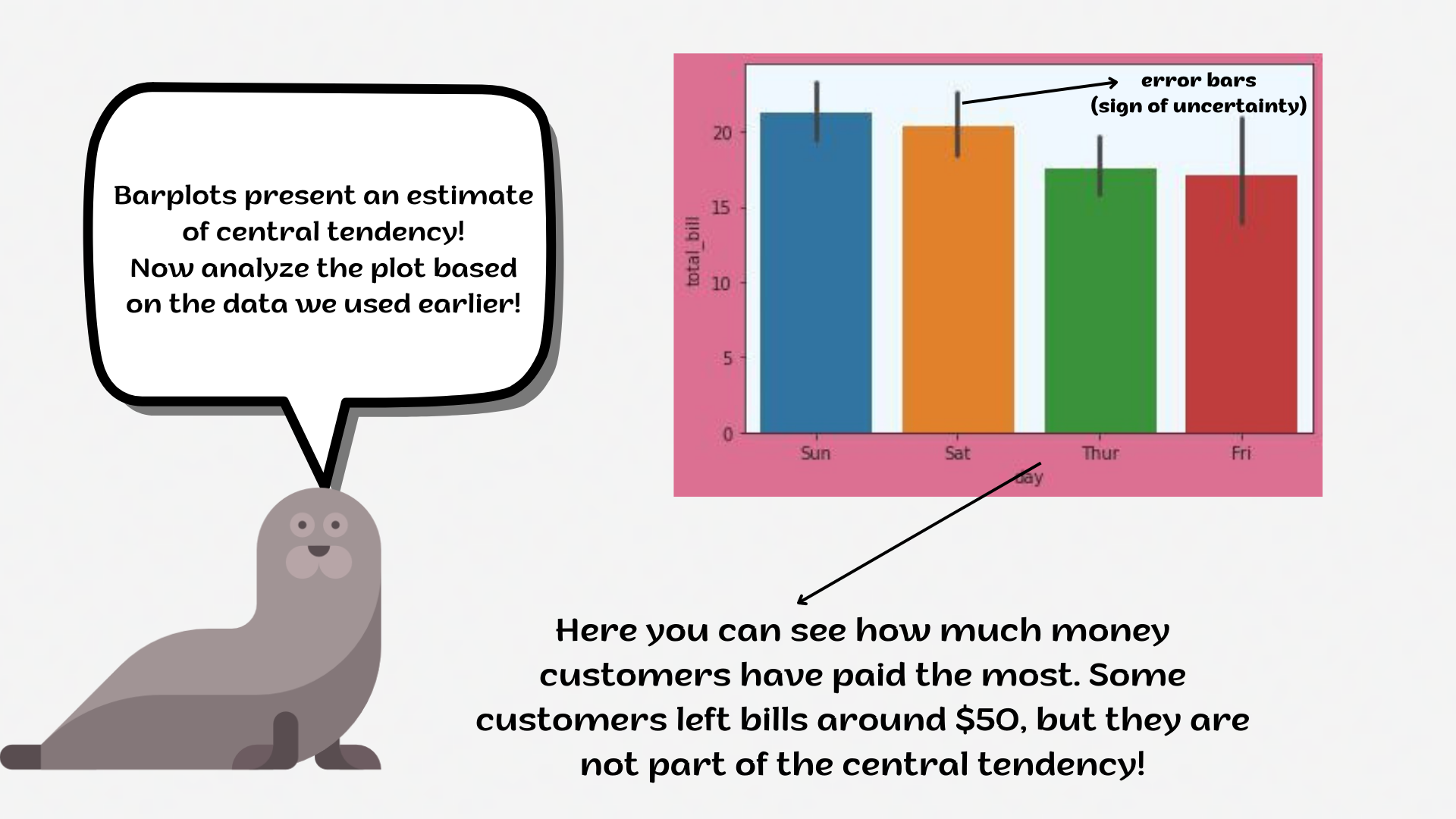Course Content

# Deep Dive into the seaborn Visualization

1. Light Start

2. Distributions of Data

3. Categorical Plot Types

4. Matrix Plots

5. Multi-Plot Grids

6. Regression Models

Deep Dive into the seaborn Visualization

##BarplotA `barplot` represents an estimate of the central tendency for a numeric variable with the height of each rectangle and provides some indication of the uncertainty around that estimate using error bars.

Bar plots include 0 in the quantitative axis range, and they are a good choice when 0 is a meaningful value for the quantitative variable, and you want to make comparisons against it.1. Set the `'ticks'` style.
2. Create the `barplot` using the `seaborn` library:
• Set the `x` parameter equals the `'day'`;
• Set the `y` parameter equals the `'total_bill'`;
• Set the `hue` parameter equals the `'smoker'`;
• Set the `linewidth` parameter equals `2.5`;
• Set the `capsize` parameter equals `0.1`;
• Set the `'pink'` `errcolor` parameter;
• Set the `'magma'` `palette`;
• Set the data;
• Display the plot.

Everything was clear?

Section 3. Chapter 5